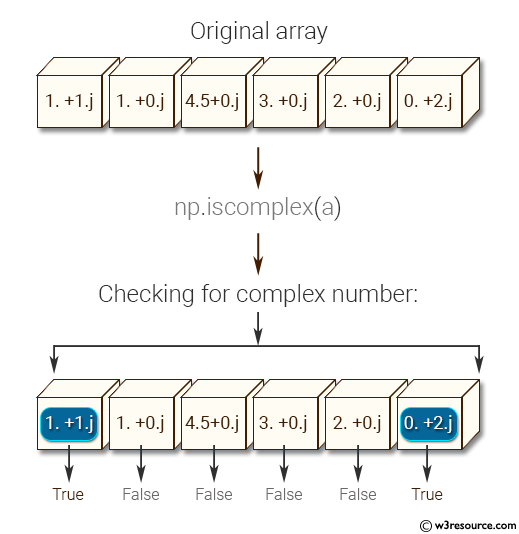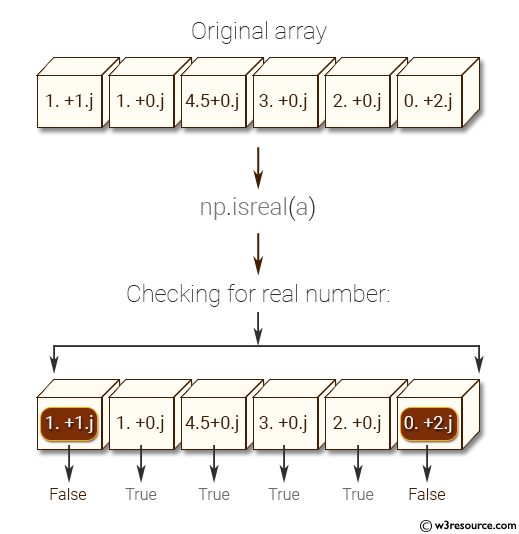﻿ NumPy: Test element-wise for complex number, real number of a given array. Also test if a given number is a scalar type or not - w3resource

# NumPy: Test element-wise for complex number, real number of a given array. Also test if a given number is a scalar type or not

## NumPy: Basic Exercise-8 with Solution

Write a NumPy program to test element-wise for complex number, real number of a given array. Also test if a given number is a scalar type or not.

Sample Solution :

Python Code :

``````import numpy as np
a = np.array([1+1j, 1+0j, 4.5, 3, 2, 2j])
print("Original array")
print(a)
print("Checking for complex number:")
print(np.iscomplex(a))
print("Checking for real number:")
print(np.isreal(a))
print("Checking for scalar type:")
print(np.isscalar(3.1))
print(np.isscalar([3.1]))
``````

Sample Output:

```Original array
[ 1.0+1.j  1.0+0.j  4.5+0.j  3.0+0.j  2.0+0.j  0.0+2.j]
Checking for complex number:
[ True False False False False  True]
Checking for real number:
[False  True  True  True  True False]
Checking for scalar type:
True
False
```

Pictorial Presentation:Python Code Editor:

Have another way to solve this solution? Contribute your code (and comments) through Disqus.

What is the difficulty level of this exercise?

﻿

Inviting useful, relevant, well-written and unique guest posts